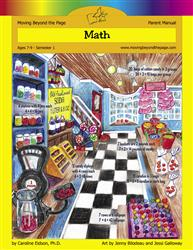# Common Core Alignment

CCSS.Math.Content.3.OA.4 - Determine the unknown whole number in a multiplication or division equation relating three whole numbers. For example, determine the unknown number that makes the equation true in each of the equations 8 × ? = 48, 5 = � ÷ 3, 6 × 6 = ?.

## 5: MathUnit 1: Multiplication and Division I
Lesson 10: More Practice With 2, 3, 4, and 5
Lesson 11: Multiples of 10
Lesson 12: Problem Solving With Multiplication
Lesson 13: What Is Division?
Lesson 14: Multiplication and Division Fact Families
Lesson 15: Unit Test
Lesson 5: Multiplication and the Abacus
Lesson 6: The Commutative Property of Multiplication
Lesson 7: Multiples of 2 and 3
Lesson 8: More Practice With 2 and 3
Lesson 9: Multiples of 4 and 5
Final Project: Multiples Mini-PostersUnit 4: Multiplication and Division II
Lesson 1: Mastering More Multiplication Facts
Lesson 12: Unit Test
Lesson 2: Multiples of 6 and 7
Lesson 3: Multiples of 8 and 9
Lesson 4: Practicing All of the Multiples
Lesson 8: Revisiting Division
Lesson 9: Multiplication and Division Facts Practice